# Subject Content

Study notes for the GCE ‘A’ level syllabus

## 07. Gravitation

[spoiler title=”Gravitational Field” open=”yes”]

• Newton’s Law of Gravitation states that the gravitational force between two point masses is directly proportional to the product of their masses and inversely proportional to the square of their separation,
i.e. $$F_G=\dfrac{Gm_1m_2}{r^2}$$ where G is the gravitational constant 6.67 $$\times$$ 10-11 N kg-2m2, m1 and m2 are the masses and r is the distance between them.
• Gravitational field strength at a point is defined as the gravitational force acting per unit mass placed at that point,
i.e. $$g=\dfrac{F_G}{m_2}=\dfrac{Gm_1}{r^2}$$ where m1 is the mass of the source of the field.
• Gravitational potential energy at a point is defined as the work done by an external agent in bringing a body from infinity to that point (without any change in kinetic energy),
i.e. $$U=-\dfrac{Gm_1m_2}{r}$$
• Gravitational potential at a point is defined as the work done per unit mass by an external agent in bringing a body from infinity to that point (without any change in kinetic energy),
i.e. $$V=-\dfrac{Gm_1}{r}$$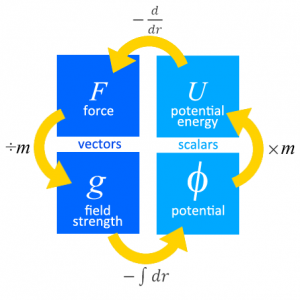[/spoiler]

[spoiler title=”Interplanetary Travel”]

• Escape speed is the minimum speed at which a body of mass m can be projected from the surface of a planet of mass M so that it reaches an infinite distance from the planet.
• By conservation of energy,
initial total energy = final total energy
$$U_i+E_{k_i}=U_f+E_{k_f}\\-\dfrac{GMm}{r}+\dfrac{1}{2}mv^2=0+0$$
$$v=\sqrt{2GM}$$

[/spoiler]

[spoiler title=”Satellite Motion”]

• For an object with mass m in orbit,
Gravitational Force = Centripetal Force
$$F_G=F_C\\\dfrac{GMm}{r^2}=mr\omega^2\\\dfrac{GM}{r^2}=r(\dfrac{2\pi}{T})^2\\\text{period, }T=2\pi\sqrt{\dfrac{r^3}{GM}}$$
• $$F_G=F_C\\\dfrac{GMm}{r^2}=\dfrac{mv^2}{r}\\\text{orbital speed, }v=\sqrt{\dfrac{GM}{r}}$$
• kinetic energy, $$E_k=\dfrac{1}{2}mv^2=\dfrac{GMm}{2r}$$
• total energy, $$E_T=E_k+U=\dfrac{GMm}{2r}-\dfrac{GMm}{r}=-\dfrac{GMm}{2r}$$

[/spoiler]

[spoiler title=”Geostationary Satellites”]

• A geostationary satellite (one that is always above the same point on the Earth) has to be in an equatorial orbit, i.e. the orbit is in the same plane as that of the equator, so that
• the centripetal force points towards the centre of the plane, and
• it moves in the same direction of rotation as the Earth, i.e. from west to east.
• It has a period of 24 h, i.e. same period as the Earth.
• It orbits with a fixed radius of 4.20 $$\times$$ 10m from the Earth’s centre.

[/spoiler]

## 06. Motion in a Circle

[accordions autoHeight=’true’]

[accordion title=”1. Rotational Kinematics”]

• Angular displacement $$\theta$$ is defined as the angle an object turns with respect to the centre of a circle. $$\theta=\dfrac{s}{r}$$ where s is the arc and r is the radius of the circle.
• One radian is the angular displacement when the arc length is equal to the radius of the circle.
• Angular velocity $$\omega$$ is defined as the rate of change of angular displacement. $$\omega=\dfrac{d\theta}{dt}$$
• For motion in a circle of fixed radius, $$\omega=\dfrac{d\theta}{dt}=\dfrac{d(\dfrac{s}{r})}{dt}=\dfrac{1}{r}\dfrac{ds}{dt}=\dfrac{v}{r}$$. Thus $$v=r\omega$$.
• Average angular velocity in one cycle. $$\omega=\dfrac{2\pi}{T}=2\pi f$$ where T is the period and f is the frequency.

[/accordion]

[accordion title=”2. Centripetal Force”]

• Centripetal acceleration $$a=\dfrac{v^2}{r} = r\omega^2$$.
• Centripetal force $$F =\dfrac{mv^2}{r} = mr\omega^2$$.

[/accordion]

[accordion title=”3. Uniform Circular Motion”]

For a body in uniform circular motion, there is a change in velocity as the direction of motion is changing. This requires an acceleration that is perpendicular to the velocity and directed towards the centre of circle. This acceleration is provided by a centripetal force. A resultant force acting on a body toward the centre of a circle provides the centripetal force.

[/accordion]

[accordion title=”4. Non-Uniform Circular Motion”]

Learn how to solve problems on circular motions of conical pendulum, cyclist, car, aircraft, swinging a pail etc.

[/accordion]

[/accordions]

## 05. Work, Energy and Power

[accordions autoHeight=’true’]

[accordion title=”1. Work Done”]

• Work done W is defined as the product of the force and the displacement made in the direction of the force.
• For a constant force F acting in same direction as the displacement s: W = F . s
• If $$\theta$$ is the angle between F and s:  $$W = F\cos\theta\cdot s$$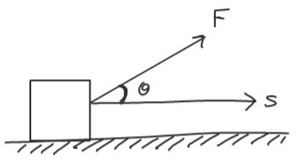• Work done = Area under F-s graph.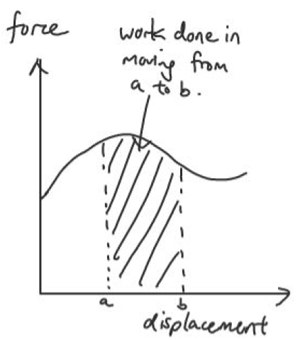[/accordion]

[accordion title=”1.1 Work Done on a Spring”]

• Work done in stretching a spring = area under F-x graph = ½ Fx = ½ kx2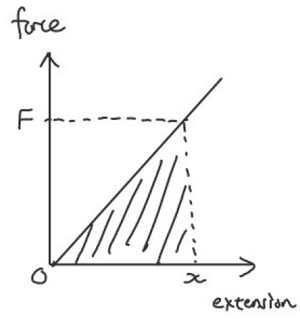[/accordion]

[accordion title=”1.2 Work Done by a Gas”]

• Work done by expanding gas = area under P-V graph = $$\int P.dV$$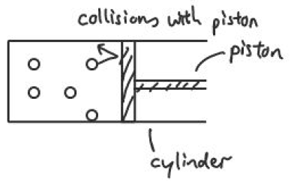[/accordion]

[accordion title=”2. Kinetic Energy”]

• Kinetic energy is the energy of a body by virtue of its motion.
• Derive kinetic energy: W = F.s = ma.s = m ½(v2 – u2)

[/accordion]
[accordion title=”3. Potential Energy”]

• Potential energy is the energy of a body by virtue of it position.
• Gravitational Potential Energy near Earth’s surface: $$E_p = mgh$$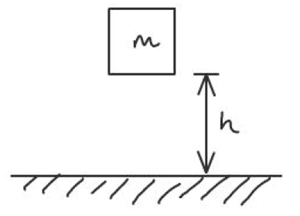[/accordion]

[accordion title=”4. Conservation of Energy”]

• The Principle of Conservation of Energy states that energy can neither be created nor destroyed in any process. It can be transformed from one form to another, and transferred from one body to another but the total amount remains constant.
• The Work-Energy Theorem states that the net work done by external forces acting on a particle is equal to the change in mechanical energy of the particle. i.e. $$W=\Delta E_k+\Delta E_p$$
• Force $$F =-\dfrac{dU}{dx} = -\text{(gradient of Potential Energy vs displacement graph)}$$

[/accordion]

[accordion title=”5. Power”]

• Power is work done per unit time
• Instantaneous Power: $$P = \dfrac{dW}{dt} = F \dfrac{ds}{dt} = F v$$,
• Average Power: $$<P> = \dfrac{W}{t} = F <v>$$

[/accordion]

[accordion title=”6. Efficiency”]

• Efficiency $$\eta= \dfrac{E_{output}}{E_{input}}\times 100 \% \text{ or} \dfrac{P_{output}}{P_{input}}\times100\%$$

[/accordion]
[/accordions]

## 15. D.C. Circuits

The Potential Divider Rule

Determining the relative brightness of light bulbs using the potential divider rule

How Internal Resistance affects brightness of light bulbs

## Electromagnetic Induction and its Effects

To explain a phenomenon that happens due to Electromagnetic Induction, we can use an acronym CFILE to structure our answer.

C stands for cutting of flux or changing of flux linkage. This is necessary for electromagnetic induction to happen. When a wire is pulled through a magnetic field perpendicular to it, it is said to be cutting the field lines. When a magnet enters a coil of wires, we can say that the magnetic flux linkage is increasing.

F stands for Faraday’s law, which states that the induced e.m.f. in a circuit is directly proportional to the rate of change of flux-linkage or to the rate of cutting of magnetic flux.

I stands for an induced current. However, do note that this is only possible if there is a closed circuit or a path for the current to flow.

L stands for Lenz’s law, which states that the direction of the induced e.m.f. is such that it tends to oppose the flux change causing it, and does oppose it if induced current flows

E stands for effect. This is really just stating how an induced current or Lenz’s law causes the phenomenon in question.

## 18. Alternating Currents

• The root-mean-square value of an alternating current is equivalent to the steady direct current that would dissipate heat at the same rate as the alternating current in a given resistor.
• For a sinusoidal source,
(a) the root mean square value of the current is given by $$I_{rms}=\frac{I_o}{\sqrt{2}}$$.
(b) the mean or average power < P > absorbed by a resistive load is half the maximum power.
$$<P>=\frac{1}{2}P_o=\frac{1}{2}{I_o}{V_o}=\frac{1}{2}{I_o}^2R =\frac{V_o^2}{2R}$$.
• An a.c. transformer is a device for increasing or decreasing an a.c. voltage. It consists of a primary coil of Np turns and voltage Vp and secondary coil of Ns turns and voltage Vs wrapped around an iron core.
• For an ideal transformer (assuming no energy is lost), the following equation is obeyed
$$\frac{N_s}{N_p}=\frac{V_s}{V_p}=\frac{I_p}{I_s}$$.
• Power loss in the transmission lines is minimized if the power is transmitted at high voltages (i.e. low currents) since $$P_{loss}=I^2R$$ where I is the current through the cables and R is the resistance of the cables.
• The equation $$P=\frac{V^2}{R}$$ is often mistakenly used to suggest that power lost is high when voltage of transmission is high. In fact, V refers to the potential difference across the cables, which often have but a fraction of the overall resistance through which the current passes.